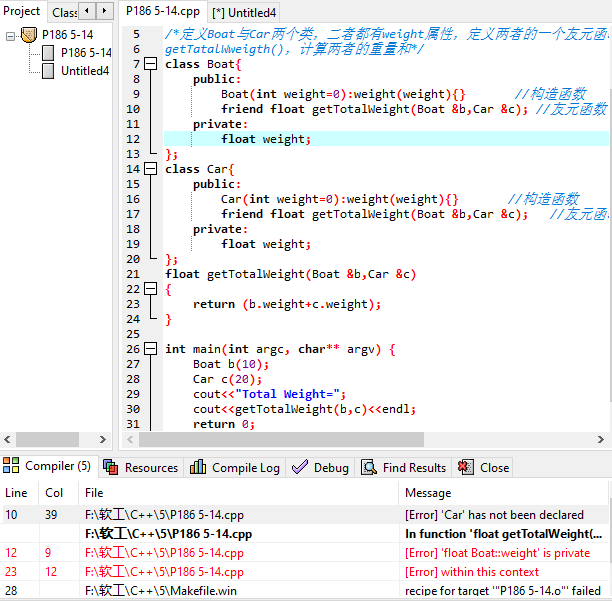``````#include <iostream>
using namespace std;
/* run this program using the console pauser or add your own getch, system("pause") or input loop */
//C++ P186 5-14
/*定义Boat与Car两个类，二者都有weight属性，定义两者的一个友元函数
getTatalWweigth()，计算两者的重量和*/
class Boat{
public:
Boat(int weight=0):weight(weight){}       //构造函数
friend float getTotalWeight(Boat &b,Car &c); //友元函数
private:
float weight;
};
class Car{
public:
Car(int weight=0):weight(weight){}       //构造函数
friend float getTotalWeight(Boat &b,Car &c);   //友元函数
private:
float weight;
};
float getTotalWeight(Boat &b,Car &c)
{
return (b.weight+c.weight);
}

int main(int argc, char** argv) {
Boat b(10);
Car c(20);
cout<<"Total Weight=";
cout<<getTotalWeight(b,c)<<endl;
return 0;
}
``````

1个回答

``````class Car;
class Boat {
public:
Boat(int weight = 0) :weight(weight) {}       //构造函数
friend float getTotalWeight(Boat& b, Car& c); //友元函数
private:
float weight;
};
class Car {
public:
Car(int weight = 0) :weight(weight) {}       //构造函数
friend float getTotalWeight(Boat& b, Car& c);   //友元函数
private:
float weight;
};
float getTotalWeight(Boat& b, Car& c);
``````
``````#include "....h"
float getTotalWeight(Boat& b, Car& c)
{
return (b.weight + c.weight);
}
``````
``````#include "....h"
#include <iostream>
using namespace std;
int main(int argc, char** argv) {
Boat b(10);
Car c(20);
cout<<"Total Weight=";
cout<<getTotalWeight(b,c)<<endl;
return 0;
}
``````